# US8909490B2 - Battery state estimation device and battery state estimation method - Google Patents

## Info

Publication number
US8909490B2
US8909490B2 US13/393,507 US201113393507A US8909490B2 US 8909490 B2 US8909490 B2 US 8909490B2 US 201113393507 A US201113393507 A US 201113393507A US 8909490 B2 US8909490 B2 US 8909490B2
Authority
US
United States
Prior art keywords
voltage
measured value
battery
secondary battery
filter
Prior art date
Legal status (The legal status is an assumption and is not a legal conclusion. Google has not performed a legal analysis and makes no representation as to the accuracy of the status listed.)
Active, expires
Application number
US13/393,507
Other versions
US20120316812A1 (en
Inventor
Shiho Umeki
Hisafumi Asai
Hideo Nakamura
Kazuhiko Tazoe
Satoshi Segawa
Current Assignee (The listed assignees may be inaccurate. Google has not performed a legal analysis and makes no representation or warranty as to the accuracy of the list.)
Nissan Motor Co Ltd
Original Assignee
Nissan Motor Co Ltd
Priority date (The priority date is an assumption and is not a legal conclusion. Google has not performed a legal analysis and makes no representation as to the accuracy of the date listed.)
Filing date
Publication date
Family has litigation
Priority to JP2010033903 priority Critical
Priority to JP2010-033903 priority
Priority to JPP2010-033903 priority
Priority to JP2011-026032 priority
Priority to JP2011026032A priority patent/JP5691592B2/en
Priority to JPP2011-026032 priority
Priority to PCT/JP2011/053534 priority patent/WO2011102472A1/en
Application filed by Nissan Motor Co Ltd filed Critical Nissan Motor Co Ltd
Assigned to NISSAN MOTOR CO., LTD. reassignment NISSAN MOTOR CO., LTD. ASSIGNMENT OF ASSIGNORS INTEREST (SEE DOCUMENT FOR DETAILS). Assignors: ASAI, HISAFUMI, NAKAMURA, HIDEO, SEGAWA, SATOSHI, TAZOE, KAZUHIKO, UMEKI, SHIHO
Publication of US20120316812A1 publication Critical patent/US20120316812A1/en
Application granted granted Critical
Publication of US8909490B2 publication Critical patent/US8909490B2/en
Active legal-status Critical Current

• 238000006243 chemical reaction Methods 0.000 claims description 32
• 230000003044 adaptive Effects 0.000 claims description 28
• 230000000694 effects Effects 0.000 description 7

## Images

•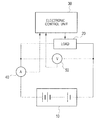•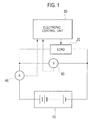•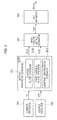•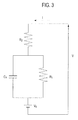•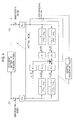•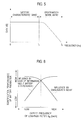•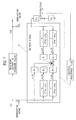•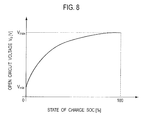•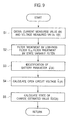•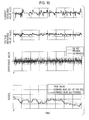••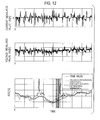•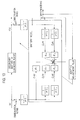•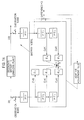## Classifications

• HELECTRICITY
• H01BASIC ELECTRIC ELEMENTS
• H01MPROCESSES OR MEANS, e.g. BATTERIES, FOR THE DIRECT CONVERSION OF CHEMICAL ENERGY INTO ELECTRICAL ENERGY
• H01M10/00Secondary cells; Manufacture thereof
• H01M10/42Methods or arrangements for servicing or maintenance of secondary cells or secondary half-cells
• H01M10/48Accumulators combined with arrangements for measuring, testing or indicating condition, e.g. level or density of the electrolyte
• H01M10/482Accumulators combined with arrangements for measuring, testing or indicating condition, e.g. level or density of the electrolyte for several batteries or cells simultaneously or sequentially
• G01R31/3624
• GPHYSICS
• G01MEASURING; TESTING
• G01RMEASURING ELECTRIC VARIABLES; MEASURING MAGNETIC VARIABLES
• G01R31/00Arrangements for testing electric properties; Arrangements for locating electric faults; Arrangements for electrical testing characterised by what is being tested not provided for elsewhere
• G01R31/36Arrangements for testing, measuring or monitoring the electrical condition of accumulators or electric batteries, e.g. capacity or state of charge [SoC]
• G01R31/382Arrangements for monitoring battery or accumulator variables, e.g. SoC
• G01R31/3842Arrangements for monitoring battery or accumulator variables, e.g. SoC combining voltage and current measurements
• G01R31/3651
• GPHYSICS
• G01MEASURING; TESTING
• G01RMEASURING ELECTRIC VARIABLES; MEASURING MAGNETIC VARIABLES
• G01R31/00Arrangements for testing electric properties; Arrangements for locating electric faults; Arrangements for electrical testing characterised by what is being tested not provided for elsewhere
• G01R31/36Arrangements for testing, measuring or monitoring the electrical condition of accumulators or electric batteries, e.g. capacity or state of charge [SoC]
• G01R31/367Software therefor, e.g. for battery testing using modelling or look-up tables
• HELECTRICITY
• H01BASIC ELECTRIC ELEMENTS
• H01MPROCESSES OR MEANS, e.g. BATTERIES, FOR THE DIRECT CONVERSION OF CHEMICAL ENERGY INTO ELECTRICAL ENERGY
• H01M10/00Secondary cells; Manufacture thereof
• H01M10/05Accumulators with non-aqueous electrolyte
• H01M10/052Li-accumulators

## Abstract

An object of the present invention is to provide a battery state estimation device and a battery state estimation method which identify parameter of a secondary battery with high accuracy. A current and a terminal voltage of secondary battery are detected, then by using the current measured value and terminal voltage measured value thus detected, the terminal voltage of secondary battery based on predetermined battery model is estimated, and the parameter of the secondary battery is identified such that a difference between terminal voltage measured value and voltage estimated value is converged to zero. In the identifying of parameter of secondary battery, the terminal voltage measured value and terminal voltage estimated value are subjected to filter treatment by low pass filter having a common high frequency breaking characteristic and the terminal voltage measured value and terminal voltage estimated value subjected to the filter treatment are used.

## Description

TECHNICAL FIELD

The present invention relates to a battery state estimation device and a battery state estimation method.

BACKGROUND ART

Japanese Patent Unexamined Publication No. 2003-185719 discloses a control device of a secondary battery as set forth below. That is, the control device of the secondary battery defines a predetermined battery model, and a current measured value and a terminal voltage measured value of the secondary battery are converted into a state quantity by using a state variable filter which is based on a battery model. By using the state quantity, the control device of the secondary battery estimates the secondary battery's terminal voltage which is based on the battery model. Then, the control device of the secondary battery identifies a parameter of the secondary battery such that a difference between the voltage measured value and the terminal voltage estimated based on the battery model is converged to zero.

SUMMARY OF INVENTION

In the Japanese Patent Unexamined Publication No. 2003-185719, however, a part of the current measured value and terminal voltage measured value of the secondary battery is used for estimating the terminal voltage without being subjected to a filter treatment by a state variable filter. Herein, the current measured value and terminal voltage measured value of the secondary battery are those measured by an ammeter or a voltmeter, therefore, ordinarily include an observation noise. Therefore, the Japanese Patent Unexamined Publication No. 2003-185719 has such a problem that an influence by the observation noise causes an insufficient accuracy of identifying the parameter of the secondary battery.

It is an object of the present invention to provide a battery state estimation device and a battery state estimation method which are capable of identifying the parameter of the secondary battery with a high accuracy.

For solving the above problem, an aspect of the present invention includes a battery state estimation device and a battery state estimation method, where a current of a secondary battery and a terminal voltage of the secondary battery are detected, then by using the current measured value and terminal voltage measured value thus detected, the terminal voltage of the secondary battery which terminal voltage is based on a predetermined battery model is estimated, and then a parameter of the secondary battery is identified such that a difference between the terminal voltage measured value and the voltage estimated value is converged to zero. In the identifying of the parameter of the secondary battery, the terminal voltage measured value and the terminal voltage estimated value are subjected to a filter treatment by a low pass filter having a common high frequency breaking characteristic, and the terminal voltage measured value and the terminal voltage estimated value which are subjected to the filter treatment are used.

According to the aspect of the present invention, in the identifying of the parameter of the secondary battery, the terminal voltage measured value and the terminal voltage estimated value are subjected to a filter treatment by a low pass filter having a common high frequency breaking characteristic, and the terminal voltage measured value and the terminal voltage estimated value which are subjected to the filter treatment are used. By this operation, the influence of the observation noise included in the current measured value and terminal voltage measured value can be effectively removed, as a result, making it possible to identify a parameter of the secondary battery with a high accuracy.

BRIEF DESCRIPTION OF DRAWINGS

FIG. 1 is a diagram showing the structure of a control system of a secondary battery according to a first embodiment.

FIG. 2 is a functional block diagram of an electronic control unit 30 according to the first embodiment.

FIG. 3 is a diagram showing an equivalent circuit model which shows a battery model of the secondary battery.

FIG. 4 is a block diagram of an adaptive identification system according to the first embodiment.

FIG. 5 is a drawing showing frequency band characteristics of a current measured value I(k), a voltage measured value V(k) and a voltage estimated value V^(k).

FIG. 6 is a relative drawing showing a relation between a cutoff frequency of a low pass filter Glpf and an influence which may be caused to an identification performance of a battery parameter by lack of information necessary for obtaining a battery characteristic and by an observation noise.

FIG. 7 is a block diagram of an adaptive identification system which is an equivalent transformation of FIG. 4.

FIG. 8 is a drawing showing an example of an open circuit voltage relative to state of charge characteristic of the secondary battery.

FIG. 9 is a flowchart showing estimation processings of the battery parameter and state of charge, according to the first embodiment.

FIG. 10 is a drawing showing simulation results of the estimation processings of the state of charge, according to the first embodiment.

FIG. 11 is a block diagram of an adaptive identification system according to a second embodiment.

FIG. 12 is a drawing showing simulation results of the estimation processings of the state of charge, according to the second embodiment.

FIG. 13 is an example of a block diagram of an adaptive identification system, according to a third embodiment.

FIG. 14 is another example of the block diagram of the adaptive identification system, according to the third embodiment.

DESCRIPTION OF EMBODIMENTS

Hereinafter, the embodiments of the present invention will be set forth based on the drawings.

First Embodiment

A control system shown in FIG. 1 is an example where a control device of a secondary battery according to the present invention is applied to a system which drives a load such as motor by a secondary battery or which charges the secondary battery by an electric power caused by a motor's regeneration or by an electric power generated by an alternator with an engine as a power source.

The secondary battery 10 has such a structure that a plurality of unit cells are connected in series. As the unit cell included in the secondary battery 10, for example, a lithium-series secondary battery such as lithium ion secondary battery is raised. As a load 20, for example, a motor and the like are raised.

A current sensor 40 is a sensor for detecting a charge-discharge current flowing through the secondary battery 10. A signal detected by the current sensor 40 is sent out to an electronic control unit 30. A voltage sensor 50 is a sensor for detecting a terminal voltage of the secondary battery 10. A signal detected by the voltage sensor 50 is sent out to the electronic control unit 30.

The electronic control unit 30 is a control unit for controlling the secondary battery 10 and includes a microcomputer which includes a CPU for operating a program, a ROM and a RAM which memorize the program or operation results, an electronic circuit, and the like.

As shown in FIG. 2, the electronic control unit 30 includes a current detector 301, a voltage detector 302, a battery parameter estimator 303, an open circuit voltage estimator 304 and an SOC estimator 305. The battery parameter estimator 303 includes a low pass filter operator 3031, a state variable filter operator 3032, and an adaptive identification operator 3033.

The current detector 301 obtains a signal from an ammeter 40 at a predetermined period and then, based on the signal from the ammeter 40, detects the charge-discharge current flowing through the secondary battery 10, to thereby obtain a current measured value I(k). The current detector 301 sends out the thus obtained current measured value I(k) to the battery parameter estimator 303.

The voltage detector 302 obtains a signal from a voltmeter 50 at a predetermined period and then, based on the signal from the voltmeter 50, detects the terminal voltage of the secondary battery 10, to thereby obtain a voltage measured value V(k). The voltage detector 302 sends out the thus obtained voltage measured value V(k) to the battery parameter estimator 303.

The battery parameter estimator 303 defines a battery model of the secondary battery 10, and then, from the current measured value I(k) detected by the current detector 301 and the voltage measured value V(k) detected by the voltage detector 302, collectively estimates a battery parameter φ^(k) of the battery model of the secondary battery 10 through an adaptive digital filter operation.

Herein, the “^” added to a right shoulder of “φ” of the φ^(k) denotes an estimated value. In FIG. 2, “^” as an estimated value is respectively disposed right above “φ” of φ(k), right above “V” of V0(k), and right above “S” of SOC(k). As shown by the following expression (1), however, these are respectively equivalent to φ^(k), V0^(k) and SOC^(k). Hereinafter, the same is true of V^(k).

$[ Expression ⁢ ⁢ 1 ] ϕ ^ ⁡ ( k ) = ϕ ^ ⁡ ( k ) V 0 ^ ⁡ ( k ) = V ^ 0 ⁡ ( k ) SOC ^ ⁡ ( k ) = S ^ ⁢ OC ⁡ ( k ) } ( 1 )$

Hereinafter, a method of estimating the battery parameter φ^(k) of the secondary battery 10 by the battery parameter estimator 303 will be set forth.

First, “battery model” used according to this embodiment will be set forth. FIG. 3 is an equivalent circuit model of the battery model of the secondary battery 10 and the equivalent circuit model shown in FIG. 3 is given by the following expression (2).

$[ Expression ⁢ ⁢ 2 ] V ⁡ ( t ) = C 1 · R 1 · R 2 · s + R 1 + R 2 C 1 · R 1 · s + 1 · I ⁡ ( t ) + V 0 ⁡ ( t ) ( 2 )$

Herein, a model input is a current I [A] (a positive value denotes charge while a negative value denotes discharge), a model output denotes a terminal voltage V [V], R1 [Ω] denotes a charge transfer resistance, R2 [Ω] denotes a pure resistance, C1 [F] denotes an electric double layer capacity, and V0 [V] denotes an open circuit voltage. In the expression (2), s denotes a differential operator. The battery model according to this embodiment is a reduction model (primary—first order) which does not specifically separate a positive electrode from a negative electrode, however, can relatively accurately show the charge-discharge characteristic of an actual battery. As stated above according to this embodiment, the structure will be set forth with an order of the battery model set to primary (first order) as an example.

Then, expressing R1, R2, C1 by the following expression (3) allows the above expression (2) to be given by the following expression (4).

$[ Expression ⁢ ⁢ 3 ] K = R 1 + R 2 , ⁢ T 1 = C 1 · R 1 , ⁢ T 2 = C 1 · R 1 · R 2 R 1 + R 2 ( 3 ) [ Expression ⁢ ⁢ 4 ] V ⁡ ( t ) = K · ( T 2 · s + 1 ) T 1 · s + 1 · I ⁡ ( t ) + V 0 ⁡ ( t ) ( 4 )$

According to this embodiment, from the battery model shown in the above expression (4), the battery parameter estimator 303 estimates the battery parameter φ^(k) of the battery model shown in FIG. 3 through the adaptive digital filter. Hereinafter, an explanation will be made about the method of estimating the battery parameter φ^(k) by the battery parameter estimator 303.

First, it is conceived that an open circuit voltage V0(t) is obtained by integrating, from an initial state, a current I(t) multiplied by a variable parameter h. In this case, the open circuit voltage V0(t) can be given by the following expression (5).

$[ Expression ⁢ ⁢ 5 ] V 0 ⁡ ( t ) = h s · I ⁡ ( t ) ( 5 )$

Then, substituting the above expression (5) into the above expression (4) gives the following expression (6) to be led to the following expression (7) after an arrangement.

$[ Expression ⁢ ⁢ 6 ] V ⁡ ( t ) = K · ( T 2 · s + 1 ) T 1 · s + 1 · I ⁡ ( t ) + h s · I ⁡ ( t ) ( 6 ) [ Expression ⁢ ⁢ 7 ] V ⁡ ( t ) = K · T 2 · s 2 + ( K + T 1 · h ) · s + h T 1 · s 2 + s · I ⁡ ( t ) ( 7 )$

The above expression (2) and above expression (7) respectively correspond to the following expression (8) and following expression (9), and correspond to those where the order of each of A(s) and B(s) is set to primary (first order) in the following expression (8) and following expression (9).

$[ Expression ⁢ ⁢ 8 ] V ⁡ ( t ) = B ⁡ ( s ) A ⁡ ( s ) · I ⁡ ( t ) + V 0 ⁡ ( t ) ( 8 ) [ Expression ⁢ ⁢ 9 ] V ⁡ ( t ) = s · B ⁡ ( s ) + A ⁡ ( s ) · h s · A ⁡ ( s ) · I ⁡ ( t ) ( 9 )$

Herein, A(s) and B(s) are each a polynomial function of s, where the A(s) and B(s) have the same order.

Then, introducing a known constant ki (i=1, 2, n) to the above expression (7) can give the following expression (10) and expression (11).

$[ Expression ⁢ ⁢ 10 ] f Vi ⁡ ( t ) = s 2 - i s 2 + k 1 · s + k 2 · V ⁡ ( t ) f Ii ⁡ ( t ) = s 2 - i s 2 + k 1 · s + k 2 · I ⁡ ( t ) } ( 10 ) [ Expression ⁢ ⁢ 11 ] y ⁡ ( t ) = ∑ i = 1 2 ⁢ ⁢ { I i ⁢ f Vi ⁡ ( t ) + b 0 ⁢ i ⁢ f Ii ⁡ ( t ) } = [ I i b 0 ⁢ i ] · [ f Vi f Ii ] ( 11 )$

In the above expression (11), Ii and b0i are each a parameter including an unknown parameter (T1, T2, K, h). fvi and fIi are each a conversion state quantity obtained by subjecting the values I(k), V(k) which are respectively measurable by the ammeter 40 and voltmeter 50 to a filter treatment by a state variable filter. Since the above expression (11) is a product sum of these, the above expression (11) coincides with the following expression (12) which is a standard form of the adaptive digital filter.
[Expression 12]
y(t)=φTω  (12)

In the above expression (12), φT=[Ii, b0i], ω=[fvi, fIi].

Then, based on an algorithm shown in the following expression (13), an identification of the battery parameter φ^(k) of the battery model is implemented from a conversion state quantity ω(k) pursuant to an adaptive adjustment rule such that a difference between a voltage estimated value V^(k) which is a terminal voltage estimated value of the secondary battery 10 estimated by the above battery model and the voltage measured value V(k) which is an actual measured value detected by the voltmeter 50 and obtained by the voltage detector 302 can be converged to zero. In this case, according to this embodiment, “both limits trace gain method” where a logical defect of a simple “adaptive digital filter by least square approach” has been improved can be used. Herein, the logical defect signifies that once the estimated value is converged, an accurate estimation cannot be accomplished again even when the parameter changes afterward.

$[ Expression ⁢ ⁢ 13 ] ϕ ^ ⁡ ( k ) = ϕ ^ ⁡ ( k - 1 ) - γ ⁡ ( k ) · Γ ⁡ ( k - 1 ) · ζ ⁡ ( k ) · e ⁡ ( k ) e ⁡ ( k ) = V ^ ⁡ ( k ) - V ⁡ ( k ) V ^ ⁡ ( k ) = ζ T ⁡ ( k ) · ϕ ^ ⁡ ( k - 1 ) } ( 13 )$

The above expression (13) includes sequential expressions for adaptively calculating the battery parameter φ^(k). γ(k) and Γ(k−1) are each an adaptive gain, of these, γ(k) is a scalar gain (error gain) while Γ(k−1) is a line gain (signal gain). Then, when a state quantity ζ(k) at a time point k is obtained by the above expression (13), it is possible to calculate e(k) which is a difference between the voltage estimated value V^(k) which is the terminal voltage estimated value of the secondary battery 10 estimated from the battery model and the voltage measured value V(k) detected by the voltmeter 50 and obtained by the voltage detector 302. Converging this e(k) to zero can sequentially calculate the battery parameter φ^(k).

Herein, according to this embodiment, as shown in FIG. 2, the battery parameter estimator 303 has the low pass filter operator 3031, the state variable filter operator 3032 and the adaptive identification operator 3033. Then, according to this embodiment, the low pass filter operator 3031, the state variable filter operator 3032 and the adaptive identification operator 3033 calculate the battery parameter φ^(k) by the method to be set forth below. Hereinafter, the method of calculating the battery parameter φ^(k) according to this embodiment will be set forth referring to a block diagram of an adaptive identification system shown in FIG. 4.

That is, according to this embodiment, for calculating the battery parameter φ^(k) according to the above method, the low pass filter operator 3031 implements the filter treatment by a low pass filter Glpf, as shown in FIG. 4. By this operation, the low pass filter operator 3031 can remove the observation noise with respect to the current measured value I(k) detected by the current detector 301 and the voltage measured value V(k) detected by the voltage detector 302.

Then, by using the current measured value I(k) and voltage measured value V(k) from which the observation noise was removed by the low pass filter Glpf, the state variable filter operator 3032 obtains the conversion state quantity ω(k) (conversion state quantities ω1(k), ω2(k), ω3(k), ω4(k), ω5(k)), using the state variable filter, as shown in FIG. 4. That is, according to this embodiment, in the above expressions (10) and (11), as I(t) and V(t), those subjected to the filter treatment which uses the low pass filter Glpf and is implemented by the low pass filter operator 3031 to thereby remove the observation noise are used. Then, based on the conversion state quantity ω(k), the state variable filter operator 3032 calculates the voltage estimated value V^(k) which is the terminal voltage estimated value based on the battery model.

Then, as shown in FIG. 4, by using the conversion state quantity ω(k) obtained by the state variable filter operator 3032 as well as the current measured value I(k) and voltage measured value V(k) from which the observation noise was removed by the low pass filter Glpf, the adaptive identification operator 3033 implements an identification of the battery parameter φ^(k) (φ1, φ2, φ3, φ4, φ5) of the battery model. That is, according to this embodiment, in the above expression (13), as V(k) and V^(k), those subjected to the filter treatment which uses the low pass filter Glpf and is implemented by the low pass filter operator 3031 to thereby remove the observation noise are used.

In this way, the current measured value I(k) and voltage measured value V(k) are subjected to the filter treatment which uses the low pass filter Glpf, to thereby remove the observation noise. By this operation, in the identification of the battery parameter φ^(k) by converging to zero the difference e(k) between the voltage estimated value V^(k) and the voltage measured value V(k), an influence by the observation noise can be effectively removed. As a result, the estimation accuracy of the battery parameter φ^(k) can be improved.

Herein, the low pass filter Glpf used according to this embodiment is not specifically limited, however, the one given by the following expression (14) may be raised.

$[ Expression ⁢ ⁢ 14 ] G lpf = s n - 1 s n + k n - 1 · s n - 1 + … + k 1 · s + k 0 ( 14 )$

Herein, as shown in FIG. 5, the current measured value I(k) and voltage measured value V(k) each includes a frequency band which is necessary for obtaining the battery characteristic, for example, a battery characteristic band (0 Hz to f1 Hz) shown in FIG. 5 and a frequency hand based on the observation noise, for example, an observation noise band (f1 Hz to f2 Hz) in the example shown in FIG. 5. The frequency band based on the observation noise is considered to be on a frequency side higher than the frequency band necessary for obtaining the battery characteristic. In addition, FIG. 5 shows the frequency band characteristic of the current measured value I(k) and voltage measured value V(k). Herein, the frequency band of the battery characteristic can be measured, for example, by cole-cole plot and the like. In addition, the frequency band based on the observation noise can be measured by FFT (fast Fourier transformation) and the like.

Then, as shown in FIG. 6, an influence by the lack of information necessary for obtaining the battery characteristic and an influence by the observation noise are in a trade-off relationship with each other, with respect an identification performance of the battery parameter relative to a cutoff frequency of the low pass filter Glpf. Thus, as the cutoff frequency of the low pass filter Glpf, it is preferable to select such a frequency that can suppress the observation noise without attenuating the frequency band necessary for obtaining the battery characteristic. Specifically, the cutoff frequency of the low pass filter Glpf used by the low pass filter operator 3031 is preferably more than or equal to the cutoff frequency of a state variable filter used by the state variable filter operator 3032, more preferably equal to the cutoff frequency of the state variable filter. By this, it is possible to extract the frequency band necessary for obtaining the battery characteristic while sufficiently reducing the observation noise. As a result, the adaptive identification operator 3033 can enhance the identification accuracy in identifying the battery parameter φ^(k) of the battery model. In addition, this is likewise applicable to the method of carrying out the filter treatment by the low pass filter Glpf on the voltage estimated value V^(k) and voltage measured value V(k) which are each an equivalent transformation of FIG. 4 (refer to FIG. 7).

In addition, according to this embodiment, it is preferable that the low pass filter Glpf having the same characteristic be used for the filter treatment of the current measured value I(k), voltage measured value V(k) and voltage estimated value V^(k). This can eliminate a phase shift, thereby enhancing the identification accuracy of the battery parameter φ^(k) of the battery model.

Then, together with the conversion state quantity ω(k), the battery parameter φ^(k) of the secondary battery 10 thus calculated is sent out to the open circuit voltage estimator 304 from the battery parameter estimator 303, as shown in FIG. 2.

The open circuit voltage estimator 304 estimates the open circuit voltage of the secondary battery 10 based on the battery parameter φ^(k) and conversion state quantity ω(k) which are calculated by the battery parameter estimator 303, to thereby calculate the open circuit voltage estimated value V0^(k). Hereinafter, the method of calculating the open circuit voltage estimated value V0^(k) will be set forth.

That is, according to this embodiment, substituting into the expression (4) the battery parameter φ^(k) calculated by the above expression (13) and the conversion state quantity ω(k) calculated by the above expression (10) calculates the open circuit voltage estimated value V0^(k).

Herein, the battery parameter φ^(k) corresponds to the parameters Ii, b0i which include the unknown parameters (T1, T2, K, h), as set forth above. Therefore, substituting into the expression (4) the battery parameter φ^(k) and conversion state quantity ω(k) which are calculated by the battery parameter estimator 303 can calculate the open circuit voltage estimated value V0^(k). The open circuit voltage estimator 304 sends out the thus calculated open circuit voltage estimated value V0^(k) to the SOC estimator 305.

From the open circuit voltage estimated value V0^(k) calculated by the open circuit voltage estimator 304, the SOC estimator 305 calculates the state of charge estimated value SOC^(k) based on a predetermined open circuit voltage relative to state of charge characteristic of the secondary battery 10. In addition, FIG. 8 shows an example of the open circuit voltage relative to state of charge characteristic of the secondary battery 10. According to this embodiment, the open circuit voltage relative to state of charge characteristic of the secondary battery 10 is memorized in advance in the RAM provided in the electronic control unit 30. The open circuit voltage relative to state of charge characteristic of the secondary battery 10 can be calculated by obtaining the relation between the open circuit voltage and the state of charge through experiments and the like implemented for the secondary battery 10.

Then, estimation processings of the battery parameter φ^(k) and state of charge estimated value SOC^(k) according to this embodiment will be set forth referring the flowchart shown in FIG. 9. Herein, the processings shown in FIG. 9 are to be implemented at a constant period, for example, every 100 msec. In the following explanation, I(k) denotes a current value of the present implementation period, that is, the present measured value, while I(k−1) denotes a current value of the previous implementation period (one time before), that is, a former measured value (one time before). Values other than the current value will be denoted likewise. In addition, the processings to be set forth below are implemented by the electronic control unit 30.

First, at step S1, the current detector 301 and voltage detector 302 respectively obtain the current measured value I(k) and voltage measured value V(k) respectively. The current measured value I(k) is sent out to the battery parameter estimator 303.

At step S2, the low pass filter operator 3031 of the battery parameter estimator 303 subjects the current measured value I(k) and voltage measured value V(k) to the filter treatment which uses the low pass filter Glpf, to thereby remove the observation noise. Then, according to the above expressions (10) and (11), the state variable filter operator 3032 of the battery parameter estimator 303 subjects the current measured value I(k) and voltage measured value V(k) (with the observation noise removed) to the filter treatment which uses the state variable filter, to thereby calculate the conversion state quantity ω(k).

At step S3, by using the conversion state quantity ω(k) calculated at step S2 and the voltage measured value V(k) with the observation noise removed, the adaptive identification operator 3033 of the battery parameter estimator 303 implements the identification of the battery parameter φ^(k) of the battery model according to the above expression (13). Herein, the voltage estimated value V^(k) used for implementing the identification of the battery parameter φ^(k) is the one that was subjected to the filter treatment which is implemented by the low pass filter operator 3031 and which uses the low pass filter Glpf.

At step S4, according to the above expressions (4), (10) and (13), the open circuit voltage estimator 304 calculates the open circuit voltage estimated value V0^(k) based on the battery parameter φ^(k) and conversion state quantity ω(k) which are calculated by the battery parameter estimator 303. Then, the thus calculated open circuit voltage estimated value V0^(k) is sent out to the SOC estimator 305.

At step S5, by using the open circuit voltage estimated value V0^(k) calculated by the open circuit voltage estimator 304, the SOC estimator 305 calculates the state of charge estimated value SOC^(k) based on the predetermined open circuit voltage relative to state of charge characteristic of the secondary battery 10.

According to this embodiment, as set forth above, the battery parameter φ^(k) of the battery model of the secondary battery 10 and the state of charge estimated value SOC^(k) of the secondary battery 10 arc estimated.

FIG. 10 shows results of verifying the effects of this embodiment by a simulation using the battery model. In FIG. 10, shown from the top are a profile showing the change of the current measured value I(k), a profile showing the change of the voltage measured value V(k), a profile showing the change of the difference e(k)=V(k)−V^(k), and a profile showing the change of the estimated value of the state of charge SOC. Then, of these, with respect to the difference e(k) and the estimated value of the state of charge SOC, those simulated by using the current measured value I(k) and voltage measured value V(k) which were subjected to the filter treatment by the low pass filter Glpf are denoted by a solid line, while those simulated by using the current measured value I(k) and voltage measured value V(k) which were not subjected to the filter treatment by the low pass filter Glpf are denoted by a dotted line. Moreover, with respect to the estimated value of the state of charge SOC, a true value is denoted by a chain line in addition to the above estimated values.

As shown in FIG. 10, when the filter treatment was not implemented by the low pass filter Glpf, the difference e(k)=V(k)−V^(k) causes a large slur, as a result, the estimated value of the state of charge SOC is deviated from the true value. On the other hand, when the current measured value I(k) and voltage measured value V(k) were subjected to the filter treatment by the low pass filter Glpf, the difference e(k)=V(k)−V^(k) was converged to zero. It can be verified that this makes it possible to preferably estimate the battery parameter, as a result, making it possible to estimate the state of charge SOC with a high accuracy.

According to this embodiment, the voltage estimated value V^(k) which is based on the battery model of the secondary battery 10 is calculated by using the current measured value I(k) and voltage measured value V(k). For removing the influence of a measurement noise included in the current measured value I(k) and voltage measured value V(k), the current measured value I(k) and voltage measured value V(k) are subjected to the filter treatment by the low pass filter Glpf. Then, the current measured value I(k) and voltage measured value V(k) which were subjected to the filter treatment are used for estimating the battery parameter φ^(k) such that the difference e(k) between the voltage measured value V(k) and the voltage estimated value V^(k) is converged to zero. By this operation, according to this embodiment, the influence of the measurement noise included in the current measured value I(k) and voltage measured value V(k) can be effectively removed, thus making it possible to easily converge the difference e(k) between the voltage measured value V(k) and the voltage estimated value V^(k) to zero. By this, the identification accuracy of the battery parameter φ^(k) can be improved. Moreover, according to this embodiment, capability of identifying the battery parameter φ^(k) with a high accuracy can enhance the estimation accuracy of each of the open circuit voltage estimated value V0^(k) and the state of charge estimated value SOC^(k). In addition, this is likewise applicable to the method of carrying out the filter treatment by the low pass filter Glpf on the voltage estimated value V^(k) and voltage measured value V(k) which are each an equivalent transformation of FIG. 4 (refer to FIG. 7).

Moreover, according to this embodiment, the cutoff frequency of the low pass filter Glpf is made more than or equal to the cutoff frequency of the state variable filter or made same as the cutoff frequency of the state variable filter. By this, the observation noise can be selectively reduced without attenuating the information necessary for obtaining the battery characteristic, specifically, the observation noise is selected from i) the information necessary for obtaining the battery characteristic included in the current measured value I(k) and voltage measured value V(k) and ii) the observation noise. Thus, the identification accuracy of the battery parameter φ^(k) can be further improved. Especially, it is so made that the cutoff frequency of the low pass filter Glpf is the same as the cutoff frequency of the state variable filter. By this, the observation noise can be suppressed to the minimum without attenuating the information necessary for obtaining the battery characteristic.

Second Embodiment

Next, an explanation will be made about a second embodiment of the present invention.

According to the second embodiment, as the low pass filter Glpf used for the filter treatment by the low pass filter operator 3031 and as the state variable filter used for the filter treatment by the state variable filter operator 3032, those not including a differentiator (differential operator) are used. Other structures are substantially the same as those according to the first embodiment.

Referring to FIG. 11, the structure of an adaptive identification system according the second embodiment will be set forth. According to the second embodiment, as the low pass filter Glpf, one that does not have the differentiator, that is, the differential operator s is used. Moreover, of the state variable filters used according to the first embodiment, one that has the differentiator, that is, s/(s2+k1·s+k2) is subjected to an application of a partial fractional breakdown, as shown by the following expression (15). By this, the state variable filter having the differentiator is rendered to be in a form that does not have the differentiator, that is, the differential operator s. By this, the adaptive identification system according to the second embodiment has the structure like that shown in FIG. 11.

$[ Expression ⁢ ⁢ 15 ] s s 2 + k 1 · s + k 2 = s ( s + k α ) ⁢ ( s + k β ) = A ( s + k α ) + B ( s + k β ) ( 15 )$

According to the second embodiment, the following effects can be taken in addition to the effects of the first embodiment.

That is, according to the second embodiment, as the low pass filter Glpf used for the filter treatment by the low pass filter operator 3031 and the state variable filter used for the filter treatment by the state variable filter operator 3032, those not including the differentiator (differential operator s) are used. By this, even a short data length can make an accurate operation, thereby further reducing the influence by the observation noise. Thus, it is possible to further improve the estimation accuracy of the battery parameter of the battery model and the estimation accuracy of the state of charge SOC.

Especially, when a high-functional CPU having an FPU function cannot be used as a CPU of the electronic control unit 30 due to reduction of cost and power consumption, it is necessary to implement an operation with integer type variables. Then, when the low pass filter Glpf or state variable filter each having a differential characteristic is calculated by the integer type variables, it is necessary to coarsely set the resolution since the dynamic range of the variable is too small. Thus, in such a case, using the low pass filter Glpf or state variable filter each having the differentiator may cause an influence to the identification performance of the battery parameter φ^(k) even by a small observation noise or may cause an error to the estimated value of each of the battery parameter and the state of charge SOC. Contrary to this, according to the second embodiment, as the low pass filter Glpf and state variable filter, those not including the differentiator (differential operator s) are used. By this, even when the high-functional CPU having the FPU function cannot be used, the influence by the observation noise can be unlikely to be caused to the identification performance of the battery parameter φ^(k), thus making it possible to enhance the estimation accuracy of the battery parameter and the estimation accuracy of the state of charge SOC.

FIG. 12 shows results of verifying the effects of the second embodiment by a simulation using the battery model. In FIG. 12, shown from the top are a profile showing the change of the current measured value I(k), a profile showing the change of the voltage measured value V(k), and a profile showing the change of the estimated value of the state of charge SOC. Then, of these, with respect to the estimated value of the state of charge SOC, a solid line denotes a case where filters not including the differentiator (differential operator s) are used as the low pass filter Glpf and state variable filter while a dotted line denotes a case where filters including the differentiator (differential operator s) are used as the low pass filter Glpf and state variable filter. Moreover, with respect to the estimated value of the state of charge SOC, a true value is denoted by a chain line in addition to the above estimated values. In addition, FIG. 12 shows simulation results obtained when the operations related to the treatment by the low pass filter Glpf and state variable filter are of the integer type and the resolution is coarsely set.

As shown in FIG. 12, when the filter having the differentiator (differential operator s) is used as the low pass filter Glpf and state variable filter, the estimated value of the state of charge SOC spread in the mid-course of tests. Contrary to this, when the filter not having the differentiator (differential operator s) is used as the low pass filter Glpf and state variable filter, the battery parameter can be preferably estimated. As a result, it can be verified that the state of charge SOC can be estimated with a high accuracy.

Third Embodiment

Next, an explanation will be made about a third embodiment of the present invention.

According to the third embodiment, as the low pass filter Glpf used for the filter treatment by the low pass filter operator 3031 of the battery parameter estimator 303 and as the state variable filter used for the filter treatment by the state variable filter operator 3032 of the battery parameter estimator 303, primary filters are to be used respectively. Other structures are substantially the same as those according to the first embodiment.

That is, according to the third embodiment, as shown by the following expression (16), a partial fractional breakdown is applied to s/(s2+k·s+k2) and l/(s2+k1·s+k2) which are each a state variable filter used according to the first embodiment.

$[ Expression ⁢ ⁢ 16 ] s s 2 + k 1 · s + k 2 = A ( s + k α ) + B ( s + k β ) 1 s 2 + k 1 · s + k 2 = C ( s + k a ) + D ( s + k b ) } ( 16 )$

Then, in the above expression (16), with ka=kα and kb=kβ, the common roots between the state variable filter and the low pass filter Glpf are bound up and put together, to thereby make a structure where the primary low pass filters are connected in parallel. Thus, the structure of the adaptive identification system can be the one shown in FIG. 13. In addition, with k1=2·√(k2), designing the low pass filter Glpf as to become a multiple root makes a structure where the primary low pass filters are connected in series. Thus, the structure of the adaptive identification system can be the one shown in FIG. 14. In addition, in FIGS. 13 and 14, ζ1(k), ζ2, ζ3(k), ζ4(k) and ζ5(k) are conversion state quantities while θ1, θ2, θ3, θ4 and θ5 are battery parameters of the battery model.

According to the third embodiment, the following effects can be taken in addition to the effects of the first embodiment.

That is, according to the third embodiment, the low pass filter operator 3031 and state variable filter operator 3032 implement the filter treatment by using the primary low pass filter as the low pass filter Glpf and state variable filter. Thus, the number of operations required for the filter treatment can be reduced. For example, in the adaptive identification system shown FIG. 4, the number of multiplications is eight and the number of additions-subtractions is four, while in the adaptive identification system shown in FIGS. 13 and 14, the number of multiplications is four and the number of additions-subtractions is two. With this, the operational load in the identification of the battery parameter can be reduced.

In addition, in the above embodiments, the current detector 301 corresponds to a current detecting means of the present invention, the voltage detector 302 corresponds to a voltage detecting means of the present invention, the low pass filter operator 3031 of the battery parameter estimator 303 corresponds to a low pass filter operating means of the present invention, the state variable filter operator 3032 of the battery parameter estimator 303 corresponds to a terminal voltage estimating means of the present invention, the adaptive identification operator 3033 of the battery parameter estimator 303 corresponds to an identifying means of the present invention, the open circuit voltage estimator 304 corresponds to an open circuit voltage estimating means of the present invention, and the SOC estimator 305 corresponds to a state of charge estimating means of the present invention.

As set forth above, although the embodiments of the present invention have been explained about, these embodiments are set forth for making it easy to understand the present invention, and therefore are not set forth for limiting the present invention. Thus, each element disclosed in the embodiments includes all design changes or equivalents that are included in the technical scope of the present invention.

INDUSTRIAL APPLICABILITY

By the battery state estimation device and battery state estimation method according to the present invention, the influence of the measurement noise included in the current measured value I(k) and voltage measured value V(k) can be effectively removed, thus making it easy to converge the difference e(k) between the voltage measured value V(k) and the voltage estimated value V^(k) to zero. By this, the identification accuracy of the battery parameter φ^(k) can be improved. In addition, capability of identifying the battery parameter φ^(k) with a high accuracy can enhance the estimation accuracy of each of the open circuit voltage estimated value V0^(k) and the state of charge estimated value SOC^(k). Thus, the battery state estimation device and battery state estimation method according to the present invention have an industrial applicability.

The entire contents of a Japanese Patent Application No. P2010-033903 with a filing date of Feb. 18, 2010 and a Japanese Patent Application No. P2011-026032 with a filing date of Feb. 9, 2011 are herein incorporated by reference.

## Claims (10)

The invention claimed is:
1. A battery state estimation device, comprising:
a current detector for detecting, as a current measured value, a current of a secondary battery;
a voltage detector for detecting, as a voltage measured value, a terminal voltage of the secondary battery;
a state variable filter operator which defines a battery model of the secondary battery, wherein by using a state variable filter which is based on the battery model, the state variable filter operator subjects to a state quantity conversion the current measured value and the voltage measured value to thereby calculate a conversion state quantity, and from the conversion state quantity, the state variable filter operator estimates, as a voltage estimated value, the terminal voltage of the secondary battery which terminal voltage is based on the battery model; and
an adaptive identification operator for identifying a parameter of the secondary battery such that a difference between the voltage measured value and the voltage estimated value is converged to zero,
wherein the adaptive identification operator subjects the voltage measured value and the voltage estimated value to a filter treatment by a low pass filter having a common high frequency breaking characteristic and calculates the difference by using the voltage measured value and the voltage estimated value which are subjected to the filter treatment by the low pass filter.
2. A battery state estimation device, comprising:
a current detector for detecting, as a current measured value, a current of a secondary battery;
a voltage detector for detecting, as a voltage measured value, a terminal voltage of the secondary battery;
a low pass filter operator for subjecting the current measured value and the voltage measured value to a filter treatment by a low pass filter having a common high frequency breaking characteristic;
a state variable filter operator which defines a battery model of the secondary battery, wherein by using a state variable filter which is based on the battery model, the state variable filter operator subjects to a state quantity conversion the current measured value and the voltage measured value which are subjected to the filter treatment by the low pass filter to thereby calculate a conversion state quantity, and from the conversion state quantity, the state variable filter operator estimates, as a voltage estimated value, the terminal voltage of the secondary battery which terminal voltage is based on the battery model; and
an adaptive identification operator for identifying a parameter of the secondary battery such that a difference between the voltage measured value subjected to the filter treatment by the low pass filter and the voltage estimated value is converged to zero.
3. The battery state estimation device according to claim 1 wherein a cutoff frequency of the low pass filter is more than or equal to a cutoff frequency of the state variable filter.
4. The battery state estimation device according to claim 3 wherein the cutoff frequency of the low pass filter is equal to the cutoff frequency of the state variable filter.
5. The battery state estimation device according to claim 1 wherein the low pass filter and the state variable filter are free from a differentiator.
6. The battery state estimation device according to claim 1 wherein the low pass filter and the state variable filter include only a serial connection or a parallel connection of a primary low pass filter.
7. A battery state estimation device, comprising:
a current detecting means for detecting, as a current measured value, a current of a secondary battery;
a voltage detecting means for detecting, as a voltage measured value, a terminal voltage of the secondary battery;
a terminal voltage estimating means which defines a battery model of the secondary battery, wherein by using a state variable filter which is based on the battery model, the terminal voltage estimating means subjects to a state quantity conversion the current measured value and the voltage measured value to thereby calculate a conversion state quantity, and from the conversion state quantity, the terminal voltage estimating means estimates, as a voltage estimated value, the terminal voltage of the secondary battery which terminal voltage is based on the battery model; and
an identifying means for identifying a parameter of the secondary battery such that a difference between the voltage measured value and the voltage estimated value is converged to zero,
wherein the identifying means subjects the voltage measured value and the voltage estimated value to a filter treatment by a low pass filter having a common high frequency breaking characteristic and calculates the difference by using the voltage measured value and the voltage estimated value which are subjected to the filter treatment by the low pass filter.
8. A battery state estimation device, comprising:
a current detecting means for detecting, as a current measured value, a current of a secondary battery;
a voltage detecting means for detecting, as a voltage measured value, a terminal voltage of the secondary battery;
a low pass filter operating means for subjecting the current measured value and the voltage measured value to a filter treatment by a low pass filter having a common high frequency breaking characteristic;
a terminal voltage estimating means which defines a battery model of the secondary battery, wherein by using a state variable filter which is based on the battery model, the terminal voltage estimating means subjects to a state quantity conversion the current measured value and the voltage measured value which are subjected to the filter treatment by the low pass filter to thereby calculate a conversion state quantity, and from the conversion state quantity, the terminal voltage estimating means estimates, as a voltage estimated value, the terminal voltage of the secondary battery which terminal voltage is based on the battery model; and
an identifying means for identifying a parameter of the secondary battery such that a difference between the voltage measured value subjected to the filter treatment by the low pass filter and the voltage estimated value is converged to zero.
9. A battery state estimation method, comprising:
detecting, as a current measured value, a current of a secondary battery;
detecting, as a voltage measured value, a terminal voltage of the secondary battery;
defining a battery model of the secondary battery;
by using a state variable filter which is based on the battery model, subjecting to a state quantity conversion the current measured value and the voltage measured value to thereby calculate a conversion state quantity;
from the conversion state quantity, estimating, as a voltage estimated value, the terminal voltage of the secondary battery which terminal voltage is based on the battery model; and
identifying a parameter of the secondary battery such that a difference between the voltage measured value and the voltage estimated value is converged to zero,
wherein the identifying of the parameter of the secondary battery such that the difference between the voltage measured value and the voltage estimated value is converged to zero includes:
subjecting the voltage measured value and the voltage estimated value to a filter treatment by a low pass filter having a common high frequency breaking characteristic, and
calculating the difference by using the voltage measured value and the voltage estimated value which are subjected to the filter treatment by the low pass filter.
10. A battery state estimation method, comprising:
detecting, as a current measured value, a current of a secondary battery;
detecting, as a voltage measured value, a terminal voltage of the secondary battery;
subjecting the current measured value and the voltage measured value to a filter treatment by a low pass filter having a common high frequency breaking characteristic;
defining a battery model of the secondary battery;
by using a state variable filter which is based on the battery model, subjecting to a state quantity conversion the current measured value and the voltage measured value which are subjected to the filter treatment by the low pass filter to thereby calculate a conversion state quantity;
from the conversion state quantity, estimating, as a voltage estimated value, the terminal voltage of the secondary battery which terminal voltage is based on the battery model; and
identifying a parameter of the secondary battery such that a difference between the voltage measured value subjected to the filter treatment by the low pass filter and the voltage estimated value is converged to zero.
US13/393,507 2010-02-18 2011-02-18 Battery state estimation device and battery state estimation method Active 2032-05-09 US8909490B2 (en)

## Priority Applications (7)

Application Number Priority Date Filing Date Title
JP2010033903 2010-02-18
JP2010-033903 2010-02-18
JPP2010-033903 2010-02-18
JP2011026032A JP5691592B2 (en) 2010-02-18 2011-02-09 Battery state estimation device
JPP2011-026032 2011-02-09
JP2011-026032 2011-02-09
PCT/JP2011/053534 WO2011102472A1 (en) 2010-02-18 2011-02-18 Battery state estimation device and method of estimating battery state

## Publications (2)

Publication Number Publication Date
US20120316812A1 US20120316812A1 (en) 2012-12-13
US8909490B2 true US8909490B2 (en) 2014-12-09

# Family

## Family Applications (1)

Application Number Title Priority Date Filing Date
US13/393,507 Active 2032-05-09 US8909490B2 (en) 2010-02-18 2011-02-18 Battery state estimation device and battery state estimation method

## Country Status (6)

US (1) US8909490B2 (en)
EP (1) EP2538233B1 (en)
JP (1) JP5691592B2 (en)
CN (1) CN102483442B (en)
MX (1) MX2012002807A (en)
WO (1) WO2011102472A1 (en)

## Cited By (2)

* Cited by examiner, † Cited by third party
Publication number Priority date Publication date Assignee Title
US20140244225A1 (en) * 2013-02-24 2014-08-28 The University Of Connecticut Battery state of charge tracking, equivalent circuit selection and benchmarking
US10664562B2 (en) * 2014-02-20 2020-05-26 Fairchild Semiconductor Corporation and University of Connecticut Battery state of charge tracking, equivalent circuit selection and benchmarking

## Families Citing this family (10)

* Cited by examiner, † Cited by third party
Publication number Priority date Publication date Assignee Title
JP5842421B2 (en) * 2010-07-20 2016-01-13 日産自動車株式会社 Battery state estimation device
WO2013111231A1 (en) * 2012-01-26 2013-08-01 カルソニックカンセイ株式会社 Battery state estimation device
DE102012013739A1 (en) * 2012-07-12 2014-05-15 Sew-Eurodrive Gmbh & Co Kg Method for determining characteristics of an energy storage and energy storage system
JP5896891B2 (en) * 2012-12-17 2016-03-30 三菱重工業株式会社 Parameter estimation apparatus, parameter estimation method, power storage system, and program
US10094881B2 (en) * 2013-02-28 2018-10-09 Dongguan Cellwise Microelectronics Co., Ltd. Battery fuel gauging system
DE102014102352A1 (en) 2014-02-24 2015-08-27 Ge Energy Power Conversion Technology Limited Battery storage system with arc fault protection, energy conversion system and protection method
KR101877145B1 (en) * 2015-06-17 2018-07-10 가부시끼가이샤 도시바 Simulation-signal generation device, simulation-signal generation method and computer readable storage medium
DE102015111015A1 (en) * 2015-07-08 2017-01-12 Dr. Ing. H.C. F. Porsche Aktiengesellschaft Method and device for locating a battery module among a plurality of mutually electrically connected battery modules of a traction battery
CN106371018B (en) * 2015-07-21 2019-05-24 上汽通用汽车有限公司 Power cell of vehicle method for diagnosing faults and equipment based on battery terminal voltage estimation
JP2017070024A (en) * 2015-09-29 2017-04-06 日立オートモティブシステムズ株式会社 Battery monitoring device

## Citations (10)

* Cited by examiner, † Cited by third party
Publication number Priority date Publication date Assignee Title
JPH05142313A (en) 1991-11-19 1993-06-08 Shikoku Electric Power Co Inc Open circuit voltage estimation device for lead-acid battery
RU2131158C1 (en) 1996-07-31 1999-05-27 Кубанский государственный технологический университет Device for automatic check-up of storage battery cells for condition
JP2003185719A (en) 2001-12-18 2003-07-03 Nissan Motor Co Ltd Device for estimating charging rate for secondary battery
US20040100227A1 (en) * 2002-11-25 2004-05-27 Nissan Motor Co., Ltd. Apparatus and method for estimating charge rate of secondary cell
CN1525592A (en) 2003-02-28 2004-09-01 日产自动车株式会社 Estimating apparatus and method of input and output enabling powers for secondary cell
RU2283504C1 (en) 2005-06-28 2006-09-10 Федеральное государственное унитарное предприятие "Производственно-конструкторское предприятие "ИРИС" Automated system for control and diagnostics of accumulator batteries
RU2328753C2 (en) 2003-12-18 2008-07-10 Эл Джи Кем, Лтд. Device and method for evaluating state of charge of battery with use of neutral network
US7521895B2 (en) 2006-03-02 2009-04-21 Lg Chem, Ltd. System and method for determining both an estimated battery state vector and an estimated battery parameter vector
US20090109046A1 (en) 2007-10-30 2009-04-30 Gm Global Technology Operations, Inc. Adaptive Filter Algorithm for Estimating Battery State-of-Age
RU2361333C2 (en) 2004-11-11 2009-07-10 Эл Джи Кем., Лтд. Galvanic element condition and parametres monitoring assessment

## Family Cites Families (4)

* Cited by examiner, † Cited by third party
Publication number Priority date Publication date Assignee Title
JP3852372B2 (en) * 2002-06-05 2006-11-29 日産自動車株式会社 Secondary battery charge rate estimation device
JP4830382B2 (en) * 2005-07-19 2011-12-07 日産自動車株式会社 Secondary battery charge rate estimation device
JP4692246B2 (en) * 2005-11-29 2011-06-01 日産自動車株式会社 Secondary battery input / output possible power estimation device
JP2009072020A (en) * 2007-09-14 2009-04-02 Calsonic Kansei Corp Internal state estimating device of secondary battery

## Patent Citations (15)

* Cited by examiner, † Cited by third party
Publication number Priority date Publication date Assignee Title
JPH05142313A (en) 1991-11-19 1993-06-08 Shikoku Electric Power Co Inc Open circuit voltage estimation device for lead-acid battery
RU2131158C1 (en) 1996-07-31 1999-05-27 Кубанский государственный технологический университет Device for automatic check-up of storage battery cells for condition
JP2003185719A (en) 2001-12-18 2003-07-03 Nissan Motor Co Ltd Device for estimating charging rate for secondary battery
US20040100227A1 (en) * 2002-11-25 2004-05-27 Nissan Motor Co., Ltd. Apparatus and method for estimating charge rate of secondary cell
CN1503399A (en) 2002-11-25 2004-06-09 日产自动车株式会社 Device and method for valuting recharge rate of cell
EP1422804B1 (en) 2002-11-25 2007-09-26 Nissan Motor Company, Limited State of charge estimating apparatus and method for a secondary cell
US20040169495A1 (en) 2003-02-28 2004-09-02 Nissan Motor Co., Ltd. Estimating apparatus and method of input and output enabling powers for secondary cell
CN1525592A (en) 2003-02-28 2004-09-01 日产自动车株式会社 Estimating apparatus and method of input and output enabling powers for secondary cell
US7583059B2 (en) 2003-12-18 2009-09-01 Lg Chem, Ltd. Apparatus and method for estimating state of charge of battery using neural network
RU2328753C2 (en) 2003-12-18 2008-07-10 Эл Джи Кем, Лтд. Device and method for evaluating state of charge of battery with use of neutral network
US8103485B2 (en) * 2004-11-11 2012-01-24 Lg Chem, Ltd. State and parameter estimation for an electrochemical cell
RU2361333C2 (en) 2004-11-11 2009-07-10 Эл Джи Кем., Лтд. Galvanic element condition and parametres monitoring assessment
RU2283504C1 (en) 2005-06-28 2006-09-10 Федеральное государственное унитарное предприятие "Производственно-конструкторское предприятие "ИРИС" Automated system for control and diagnostics of accumulator batteries
US7521895B2 (en) 2006-03-02 2009-04-21 Lg Chem, Ltd. System and method for determining both an estimated battery state vector and an estimated battery parameter vector
US20090109046A1 (en) 2007-10-30 2009-04-30 Gm Global Technology Operations, Inc. Adaptive Filter Algorithm for Estimating Battery State-of-Age

## Non-Patent Citations (1)

* Cited by examiner, † Cited by third party
Title
Chinese Office Action dated Oct. 10, 2013, (4 pgs.).

## Cited By (2)

* Cited by examiner, † Cited by third party
Publication number Priority date Publication date Assignee Title
US20140244225A1 (en) * 2013-02-24 2014-08-28 The University Of Connecticut Battery state of charge tracking, equivalent circuit selection and benchmarking
US10664562B2 (en) * 2014-02-20 2020-05-26 Fairchild Semiconductor Corporation and University of Connecticut Battery state of charge tracking, equivalent circuit selection and benchmarking

## Also Published As

Publication number Publication date
CN102483442B (en) 2014-05-07
EP2538233B1 (en) 2018-08-29
CN102483442A (en) 2012-05-30
WO2011102472A1 (en) 2011-08-25
JP5691592B2 (en) 2015-04-01
MX2012002807A (en) 2012-04-02
JP2011191291A (en) 2011-09-29
US20120316812A1 (en) 2012-12-13
EP2538233A1 (en) 2012-12-26
EP2538233A4 (en) 2016-10-19

## Similar Documents

Publication Publication Date Title
Duong et al. Online state of charge and model parameters estimation of the LiFePO4 battery in electric vehicles using multiple adaptive forgetting factors recursive least-squares
Zheng et al. Influence of different open circuit voltage tests on state of charge online estimation for lithium-ion batteries
Xu et al. A new method to estimate the state of charge of lithium-ion batteries based on the battery impedance model
Li et al. A comparative study of state of charge estimation algorithms for LiFePO4 batteries used in electric vehicles
Sun et al. A novel dual-scale cell state-of-charge estimation approach for series-connected battery pack used in electric vehicles
Xiong et al. A novel method to obtain the open circuit voltage for the state of charge of lithium ion batteries in electric vehicles by using H infinity filter
Wang et al. Modeling and state-of-charge prediction of lithium-ion battery and ultracapacitor hybrids with a co-estimator
Xing et al. State of charge estimation of lithium-ion batteries using the open-circuit voltage at various ambient temperatures
US9454888B2 (en) Advanced battery early warning and monitoring system
Rahimi-Eichi et al. Online adaptive parameter identification and state-of-charge coestimation for lithium-polymer battery cells
Gholizadeh et al. Estimation of state of charge, unknown nonlinearities, and state of health of a lithium-ion battery based on a comprehensive unobservable model
He et al. State of charge estimation for electric vehicle batteries using unscented kalman filtering
Weng et al. On-board state of health monitoring of lithium-ion batteries using incremental capacity analysis with support vector regression
EP2700966B1 (en) Apparatus and method for estimating battery state
CN103907030B (en) The estimation unit of battery charge rate and the method for estimation of battery charge rate
He et al. Online estimation of model parameters and state-of-charge of LiFePO4 batteries in electric vehicles
Hentunen et al. Time-domain parameter extraction method for Thévenin-equivalent circuit battery models
Weng et al. A unified open-circuit-voltage model of lithium-ion batteries for state-of-charge estimation and state-of-health monitoring
Xiong et al. A data-driven based adaptive state of charge estimator of lithium-ion polymer battery used in electric vehicles
EP2618168B1 (en) Device for parameter estimation by means of a filter
Xia et al. A novel method for state of charge estimation of lithium-ion batteries using a nonlinear observer
Kim The novel state of charge estimation method for lithium battery using sliding mode observer
CN104285157B (en) The charged state estimation unit of battery
US8664960B2 (en) Charged state estimating device and charged state estimating method of secondary battery
CN103472403B (en) A kind of electrokinetic cell SOC compound method of estimation based on PNGV equivalent-circuit model

## Legal Events

Date Code Title Description
AS Assignment

Owner name: NISSAN MOTOR CO., LTD., JAPAN

Free format text: ASSIGNMENT OF ASSIGNORS INTEREST;ASSIGNORS:UMEKI, SHIHO;ASAI, HISAFUMI;NAKAMURA, HIDEO;AND OTHERS;SIGNING DATES FROM 20120411 TO 20120413;REEL/FRAME:028129/0087

STCF Information on status: patent grant

Free format text: PATENTED CASE

MAFP Maintenance fee payment

Free format text: PAYMENT OF MAINTENANCE FEE, 4TH YEAR, LARGE ENTITY (ORIGINAL EVENT CODE: M1551)

Year of fee payment: 4